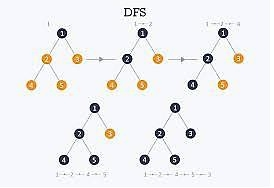# Depth First Search or DFS for a Graph

The Depth First Search (DFS) is a graph traversal algorithm. In this algorithm one starting vertex is given, and when an adjacent vertex is found, it moves to that adjacent vertex first and try to traverse in the same manner.It moves through the whole depth, as much as it can go, after that it backtracks to reach previous vertices to find new path.

To implement DFS in iterative way, we need to use the stack data structure. If we want to do it recursively, external stacks are not needed, it can be done internal stacks for the recursion calls.

Input: The Adjacency matrix of a graph.

  A B C D E F
A 0 1 1 1 0 0
B 1 0 0 1 1 0
C 1 0 0 1 0 1
D 1 1 1 0 1 1
E 0 1 0 1 0 1
F 0 0 1 1 1 0

Output: DFS Traversal: C F E B D A

## Algorithm

### dfs(vertices, start)

Input − The list of all vertices, and the start node.

Output − Traverse all nodes in the graph.

Begin
initially make the state to unvisited for all nodes
push start into the stack
while stack is not empty, do
pop element from stack and set to u
display the node u
if u is not visited, then
mark u as visited
for all nodes i connected to u, do
if ith vertex is unvisited, then
push ith vertex into the stack
mark ith vertex as visited
done
done
End

## Example

#include<iostream>
#include<stack>
using namespace std;
#define NODE 6
typedef struct node{
int val;
int state; //status
}node;
int graph[NODE][NODE] = {
{0, 1, 1, 1, 0, 0},
{1, 0, 0, 1, 1, 0},
{1, 0, 0, 1, 0, 1},
{1, 1, 1, 0, 1, 1},
{0, 1, 0, 1, 0, 1},
{0, 0, 1, 1, 1, 0}
};
void dfs(node *vertex, node start){
node u;
stack<node> myStack;
for(int i = 0; i<NODE; i++){
vertex[i].state = 0;//not visited
}
myStack.push(start);
while(!myStack.empty()){
//pop and print node
u = myStack.top();
myStack.pop();
cout << char(u.val+'A') << " ";
if(u.state != 1){
//update vertex status to visited
u.state = 1;
vertex[u.val].state = 1;
for(int i = 0; i<NODE; i++){
if(graph[i][u.val]){
if(vertex[i].state == 0){
myStack.push(vertex[i]);
vertex[i].state = 1;
}
}
}
}
}
}
int main(){
node vertices[NODE];
node start;
char s;
for(int i = 0; i<NODE; i++){
vertices[i].val = i;
}
s = 'C';//starting vertex C
start.val = s-'A';
cout << "DFS Traversal: ";
dfs(vertices, start);
cout << endl;
}

## Output

DFS Traversal: C F E B D A